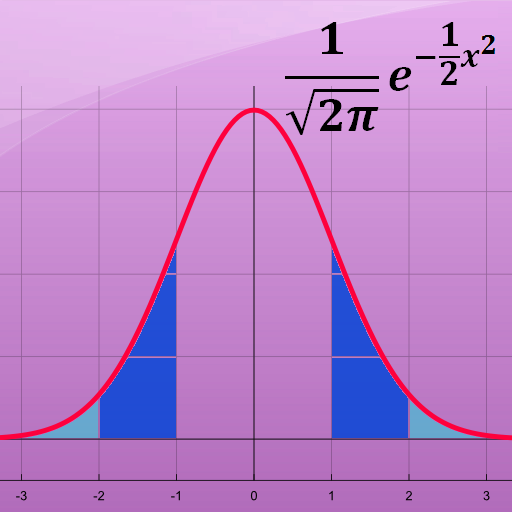# Statistics Calculator

نسخه ۲.۹
۵۰
نصب فعال
آموزش
دسته
۹۵
کیلوبایت
حجم

This free math app is a statistical calculator with various functions:

- Statistics: You are able to calculate the mean, median, variance, maximum and minimum for a set of numbers.

- Statistical Distributions: You are able to calculate the values of different Statistical Distributions. The following distributions are available: Binomial Distribution, Normal Distribution, Students t-Distribution, F-Distribution, Exponential Distribution, Poisson Distribution, Chi Squared Distribution

- Frequency Table: You are able to create a frequency table for a list of numbers. Just enter the numbers, separated by comma.

Best mathematical tool for school and college! If you are a student, it will helps you to learn statistics and probability theory.